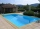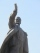# Metal tube

Calculate the metal tube mass 8dm long with the outer radius 5cm and the inner radius 4.5cm and 1cm3 of this metal is 9.5g.

Result

m =  11.341 kg

#### Solution:Leave us a comment of example and its solution (i.e. if it is still somewhat unclear...):Be the first to comment!#### To solve this verbal math problem are needed these knowledge from mathematics:

Do you know the volume and unit volume, and want to convert volume units? Do you want to convert mass units?

## Next similar examples:

1. Axial sectionAxial section of the cone is an equilateral triangle with area 208 dm2. Calculate the volume of the cone.
2. CuboidCuboid with edge a=16 cm and body diagonal u=45 cm has volume V=11840 cm3. Calculate the length of the other edges.
3. Lateral surface areaThe ratio of the area of the base of the rotary cone to its lateral surface area is 3: 5. Calculate the surface and volume of the cone, if its height v = 4 cm.
4. CubesOne cube is inscribed sphere and the other one described. Calculate difference of volumes of cubes, if the difference of surfaces in 257 mm2.
5. SphereThe surface of the sphere is 2820 cm2, and weight is 71 kg. What is its density?
6. Three tributariesIt is possible to fill the pool with three tributaries. The first would take 12 hours, the second 15 hours, and the third 20 hours. The day before the summer season began, the manager opened all three tributaries simultaneously. How long did it take to fil
7. PoolIf water flows into the pool by two inlets, fill the whole for 8 hours. The first inlet filled pool 6 hour longer than second. How long pool take to fill with two inlets separately?
8. PoolThe swimming pool is 10 m wide and 8 m long and 153 cm deep. How many hectoliters of water is in it, if the water is 30 cm below its upper edge?
9. TV transmitterThe volume of water in the rectangular swimming pool is 6998.4 hectoliters. The promotional leaflet states that if we wanted all the pool water to flow into a regular quadrangle with a base edge equal to the average depth of the pool, the prism would have.
10. Cube in a sphereThe cube is inscribed in a sphere with volume 6116 cm3. Determine the length of the edges of a cube.
11. Transforming cuboidCuboid with dimensions 8 cm, 13, and 16 cm is converted into a cube with the same volume. What is its edge length?
12. PipesWater pipe has a cross-section 1087 cm2. An hour has passed 960 m3 of water. How much water flows through the pipe with cross-section 300 cm2 per 9 hours if water flow same speed?
13. Sphere VSFind the surface and volume of a sphere that has a radius of 2 dm.The radius of the sphere we reduce by 1/3 of the original radius. How much percent does the volume and surface of the sphere change?The rectangular cuboid has a surface area 5334 cm2, its dimensions are in the ratio 2:4:5. Find the volume of this rectangular cuboid.If the heights of two cylindrical drums are in the ratio 7:8 and their base radii are in the ratio 4:3. What is the ratio of their volumes?A sculptor is duplicating a statue based on an original statue. If the scale factor of the replication is 3.2, will the new statue be larger or smaller than the original statue (enter 1 = larger, 0= smaller)